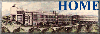# 2016-17 Handbook for Physics and Astronomy Graduate Students

## Research Programs

### Theoretical Nuclear Physics

Nuclei are prime examples of fermion many-body systems of interacting neutrons and protons. Light nuclei on the other hand fall in the category of few-body problems.

Nuclei can also be used to test aspects of elementary particle theory. For example, the nature of the neutrino can be studied via double beta decay of certain heavy nuclei. One very exciting research area involves heavy ion collisions at high energies. When two relativistic nuclei collide, hadronic matter at very high temperatures and density can be produced, possibly resulting in new forms of matter such as a quark-gluon plasma or a condensate of pi-mesons, as they may have existed in the initial stages of the universe after the big bang.

Professor Willem Kloet

From high energy experiments the substructure of nucleons in terms of quarks and gluons is evident. On the other hand at low energy a description of nuclei with nucleon degrees of freedom is prefered. How should nuclear processes in the transition region be modeled? For example the annihilation of nucleons and antinucleons into mesons is inherently a very short range process at any energy and models based on nucleon degrees of freedom are inappropriate. By constructing models for this annihilation process using other degrees of freedom, one can get insight in the substructure of the nucleon for relatively low energy processes.

Professor Aram Mekjian

My main research interests are in the areas of nuclear and particle physics and nuclear astrophysics. I also have an interest in biophysics, statistical mechanics and discrete mathematics. I have done some work in cosmology.

Presently I am writing a textbook:
Statistical Models and Stochastic Networks in Physics, Biology and Mathematical Finance.

Specific topics of interest / Present main research topics are as follows.

• Feshbach resonances in strongly correlated fermionic systems
In particular, proton/neutron systems with underlying isospin symmetries.
Unitary limit and studies of limiting universal thermodynamics in these systems
• Applying density functional theory to study properties of hadronic matter at finite temperature
• Symmetry potential and the isospin structure of neutron/proton systems and its role in neutron stars and nuclear astrophysics
• Using methods from discrete mathematics and combinatorial analysis to develop exactly soluble models in statistical physics. B Example- obtaining the canonical partition function from a recursive combinatorial approach which showed that the liquid/gas phase transition in a two component nuclear system was first order
• Random processes, Levy distributions and Polya theory - applications to physics, biology and mathematical finance
Recent and ongoing research:
• Liquid-Gas phase transition in finite two component nuclear systems - role of surface energy, symmetry energy, Coulomb energy and velocity dependence of the nuclear interaction. nucleon effective mass effects
• Multifragmentation & cluster production in nuclear collisions; also its relation to percolation theory, Ising models and Anderson localization
• Disorder effects in a nuclear systems
• Quantum critical behavior in correlated hadronic systems
• Particle multiplicity distributions in very high energy elementary particle collisions - parallels with Glauber's photon count distributions. Feynman-Wilson gas model
• Relativistic heavy ion collisions developed a statistical model
• Large scale structure in cosmology - theory of voids and hierarchical structure approaches; correlation functions
• Population genetics & reformulation of physics techniques to issues related to biophysics; stochastic abundance models
• Understanding power laws, self similarity and scale invariance in the natural sciences. Power laws from a generalized hypergeometric model.

Professor Larry Zamick

My recent and current research topics include:
I have constructed a model which consists of simply setting all T=0 two-body interaction matrix elements to zero and keeping those with T=1 as they were. This model leads to partial dynamical symmetries and corresponding degeneracies, which we have attempted to explain. Surprisingly this model gives fairly reasonable spectra for even-even nuclei in the f-p shell. We find that the restoration of the T=0 matrix elements is needed to explain staggering of high spin states in odd-even nuclei, the isovector scissors mode strengths, and to bring the nuclei somewhat away from the vibrational limit and towards the rotational limit.
It has been often said that states of different isospins have nothing to do with each other but this is not true--there is the constraint of orthogonality. Exploiting this fact I am able to greatly simplify the expression for the number of J=0 pairs in a mixed system of neutrons and protons.
I have constructed a new topic"Companion Problems in Isospin and Quasispin" in which I note that the mathematics that is used for a system of identical nucleons (e.g. only neutrons) can be used for a different problem which involves neutrons and protons (e.g. diagonalizing a six-j symbol). In the identical particle case this leads to a quasispin result concerning the number of states of a given seniority. In the companion case of mixed neutrons and protons it leads to the above mentioned simplification of the expression for the number of J=0 pairs.
The general interest in the field of nuclear structuure has shifted to nuclei far from stability--either proton rich or neutron rich--, with a mind to understanding how the heavy elements were originally formed. I have been collaborating on the magnetic moment measurements of excited states , both with the local experimental group and one from Bonn, and we are indeed going to heavy unstable nuclei e.g. 68Ge and we are planning to go further.